Pathways To Learning... Since 2005 Hong Kong Registered School 566985

# G - gallon to g-statistics - Mathematics Dictionary

A   B   C   D   E   F   G   H   I   J   K   L   M   N   O   P   Q   R   S   T   U   V   W   X   Y   Z

gallon: A number of related pre-SI units of volume (or capcity).

gambler's ruin: A number of related concepts and results in probability that is not in the gamblers' favour. Including how a player with a smaller stake goes bankrupt with a higher probability than a player with larger stake (i.e. a player against the house), even in the case of a fair game (which is often not the case); or that any player of finite wealth who only raises or maintain the same level of bets will ventually be bankrupt.

gambling odds: A method of representing payoffs of an event where x:y means a bet of y would return a profit (not winnings) of x.

game theory: In The mathematical study of mathematical objects called games which consists of a set of players, a set of actions available to players during specified stages of the game and specified method for determining the payoffs for the players.

gamma distribution: A probability distribution for a continuous random variable with semi-infinite support with the probability density function defined bywith Γ(k) being the gamma function.

gamma function: A function related to the factorial function defined by the integral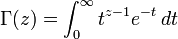Gaussian curvature: A measure of the curvature of a surface which is independent of the coordinate system in use.

Gaussian distribution: Another class="d-title" name (class="d-title" named after Carl Friedrich Gauss) for the normal distribution.

Gaussian elimination: An algorithm for finding the inverse of an invertible matrix through elementary matrix operations. Thus it is also a method for solving a system of linear equations, since such a system can be expressed as a matrix equation, which can be solved by finding the inverse of the matrix.

Gaussian integer: The complex number analogue of integers in the sense that the set is closed under addition and multiplication. They are dfined as complex numbers whose real and imaginary parta are both real numbers.

Gauss' theorem: An archaic class="d-title" name for the Divergence Theorem in vector calculus.

GCD: Also known as HCF. GCD stands for greatest common divisor(of 2 or more numbers)..

generalized eigenvalue: Given a vector which is transformed to two parallel vectors, the ratio of the magnitude of the vectors is the generalized eigenvalue. This generalizes the idea of an eigenvalue being the ratio of magnitude between the vectors before and after a transformation, which can also be thought of as the vector going through the aforementioned transformation as well as the identity transformation.

Given two matrices M1 and M2 of the same dimensions, the generalized eigenvalue λ, associated with the eigenspace containing the generalized eigenvector v, is the scalar quantity such that M1v = λM2v.

generalized linear models: A generalisation unifying various types of regressions.

general solution: Solutions of differential equations where arbitrary constants are not (or not yet) detemrined.

general term: An expression for all the terms of a sequence or a series whose value is dependent on the position of the term within the sequence or series only.

generator: Several relate concepts where a set of mathematical objects considered to be simpler than and completely specifies the mathematical object in question.

geometric distribution: The discrete probability distribution of 2 closely related situations:

1. The number of attempts, X, before the first success (in a Bernoulli trial) appears.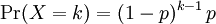2. The number of failures, Y, before the first success (in a Bernoulli trial) appears.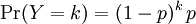Note that X = Y + 1.

geometric figure: A set of points considered as a single mathematical object with tangible attributes (such that length, distance, shape etc.).

geometric mean: Given a set of numbers, the value which, if raised to power of the number of numbers within the set, would be the same as the product of the set of numbers. Compare this to the arithmetic mean.

geometric progression A sequence where each term (except for the first) is of a fixed proportion of the previous term, that is, a constant multiple of each term would be the same as the next term.

The general term for a geometric sequence can be found by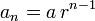where a (also known as a1) is the first term of the sequence, r is the common ratio (the fixed proportion/multiple), an is the term in question and n is the position of the term.

geometric series: The sum of the terms of a geometric progression.

The sum can be found by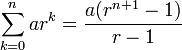for any geometric series which is not also an arithmetic series (i.e. r = 1).

In the case of an infinite geometric series (where there are infinitely many terms), the sum (strictly speaking the limit of the partial sums) is found by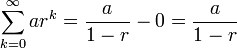geometric solid: A three-dimensional geometric figure.

geometry: A branch of mathematics concerning distances between points, sets of points or angles formed by them and ideas derived from these concepts such as adjacency, areas and shapes etc.

glide: To translate, also to slide. The term is supposed to elicit a sense of translation without rotation (or indeed any other transformations).

global maximum, global max: Also known as an absolute maximum, the greatest value over the entire domain of a function.

global minimum, global min: Also known as an absolute minimum, the least value (most negative, if relevant) over the entire domain of a function.

golden ratio: Given two quantities a and b, the ratio which is the same for both, the sum to the larger quantity and the larger to the smaller quantity.It can be shown to be described exactly by the surd (and approximately by the decimal expansions)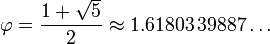golden rectangle: A rectangle whose dimensions (length and width) are in the ratio of the golden ratio.

goodness of fit: A measure of how well a set of observations agree with a proposed statistical model.

googol: A class="d-title" name for the value 10100.

googolplex: A class="d-title" name for the value 10googol.

grade: A system of measuring angles where one degree is to 1/100 of a right angle.

gradient: 1. The steepness of a slope expressed as the ratio of vertical change to horizontal change.

2. The result of applying the differential operator del, represented by the nabla symbolto find the gradient of a multi-variate scalar function.or alternatively,gram: A unt of mass within the SI system of units. Note that it is not an SI base unit - the SI base unit for mass is the kilogram.

graph: 1. A graphical illustration of the relationship of two or more variables through sets of points identified.

2. In graph theory, a set of points (vertices) together with a set of pair of points (edges).

graphical solution: A process of finding approximations of solutions through the use of graphs.

gravitation: The interaction of objects where each experience a force towards the other object proportional to their masses and inversely proportional to the square of the distance between the 2 objects.

gravitational constant: The coefficient used in the equation where force is proportional to the masses of the 2 objects concenred and inversely proportional to the square of the distance between the 2 objects - due to our choice of SI units in measuring mass and distance (and force).

gravity: See gravitation.

great circle: The largest possible circle on a sphere. For 2 points on a sphere not anti-podal to each other, the paths (along the surface) shortest and longest (without changing directions) form a great circle.

greatest common divisor: Usually abbreviated as GCD, it is also known as the highest common factor.

greatest integer function: Also known informally as the floor function.

greatest lower bound of a set, GLB: Also known as the infimum.

gross: A value before deductions allowed.

group: An algebraic structure where a set of elements is given an associative binary operation, where the set is closed under the operation; an identity element for the operatione exists and an inverse element exists.

grouped data: A representation of data by mapping each data point to exactly one of the non-intersecting categories (groups), such that only the number of occurrence (frequency) in each group is presented, ignoring difference between data points which fall under the same group.

A   B   C   D   E   F   G   H   I   J   K   L   M   N   O   P   Q   R   S   T   U   V   W   X   Y   Z